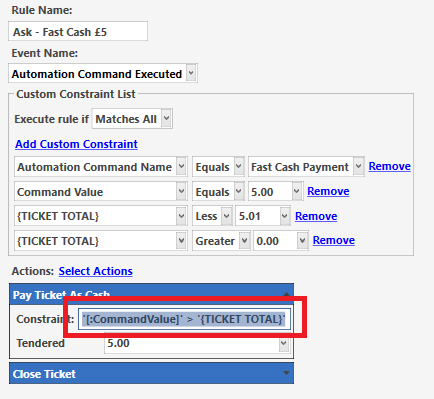# Help with constraint expression

I need help with the expression in an action within a rule. I have changed my fast cash payments buttons to be inside an ask question. I only want them to work when the fast payment button value is greater than the ticket total.

For example when the £5 fast cash button is press the ticket total must be lower than £5

im not sure on the formatting, but this is what ive tried but obviously isnt working as im not sure of the correct format

`'[:CommandValue]' > '{TICKET TOTAL}'`You cannot compare `'strings'` with a math operator.

``````[=TN('[:CommandValue]')] > [=TN('{TICKET TOTAL}')]
``````

This probably works too:

``````[=TN('[:CommandValue]') > TN('{TICKET TOTAL}')]
``````
1 Like

what would i use for greater than or equal to? `> OR =`

actually it was just that easy:

`[=TN('[:CommandValue]')] > OR = [=TN('{TICKET TOTAL}')]`

This is the correct way:

``````>=
``````

So:

``````[=TN('[:CommandValue]')] >= [=TN('{TICKET TOTAL}')]
``````

I would be shocked if that really worked.

1 Like

it did lol ill change it to >= though

I really do not think it was working. I mean I cant see how it would. I think your Rule Custom constraints were doing most of the job.

1 Like